### Gravitation - Solutions

CBSE class IX Science

NCERT Solutions
Chapter 10
Gravitation

(Page No. 134)

1. State the universal law of gravitation.

Ans. According to Newton’s universal law of gravitation:

Every mass in this universe attracts every other mass with a force which is directly proportional to the product of two masses and inversely proportional to the square of the distance between them.

i.e  $F\propto \frac{{M}_{1}{M}_{2}}{{r}^{2}}$

Or

F=$G\frac{{M}_{1}{M}_{2}}{{r}^{2}}$

2. Write the formula to find the magnitude of the gravitational force between the earth and an object on the surface of the earth.

Ans. The formula to find the magnitude of the gravitational force between the earth and an object on the surface of the earth is given below: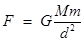F = magnitude of gravitational force
G = Universal gravitation constant

=$6.66×{10}^{-11}N{m}^{2}K{g}^{-2}$

M = mass of earth
m = mass of the object
d = distance of the object from the center of the earth

(Page No. 136)

1. What do you mean by free fall?

Ans. It is the object falling towards earth under the influence of the attractive force of earth (gravitational force) or gravity.

2. What do you mean by acceleration due to gravity?

Ans. During free fall any object that has mass experiences gravitational force towards the center of the earth and it produces acceleration in the motion of an object and that is due to gravity. so acceleration experienced by an object in its free fall is called acceleration due to gravity.” It is denoted by g.

(Page No. 138)

1. What are the differences between the mass of an object and its weight?

Ans.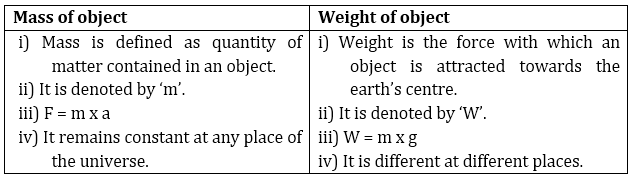2. Why is the weight of an object on the moon 1/6th its weight on the earth?

Ans. since we know
W = m x g
Mass of object remains the same whether on earth or moon but the value of acceleration on the moon is 1/6th of the value of acceleration on earth. Because of this weight of an object on the moon is 1/6th its weight on the earth.

(Page No. 141)

1. Why is it difficult to hold a school bag having a strap made of a thin and strong string?

Ans. It is difficult to hold a school bag having a strap made of a thin and strong string because a bag of that kind will make its weight fall over a small area of the shoulder and produce a greater pressure that makes holding the bag difficult and painful.

F = mg(weight)

$P\left(Pressure\right)=\frac{F\left(Weight\right)}{Area}$

2. What do you mean by buoyancy?

Ans. It is the upward force experienced by an object when it is immersed into a fluid.

3. Why does an object float or sink when placed on the surface of the water?

Ans.  As an object comes in contact with the surface of a fluid it experiences two types of forces: gravitational force or gravity that pulls the object in a downward direction and the second force is the force of buoyancy that pushes the object in the upward direction.
It is these two forces that are responsible for an object to float or sink

i.e. if gravity >buoyancy then object sinks

if gravity <buoyancy then object floats

(Page No. 142)

1. You find your mass to be 42 kg on a weighing machine. Is your mass more or less than 42 kg?

Ans. Mass will be slightly more than 42 kg.

2. You have a bag of cotton and an iron bar, each indicating a mass of 100 kg when measured on a weighing machine. In reality, one is heavier than other. Can you say which one is heavier and why?

Ans. The bag of cotton is heavier since volume of cotton bag is greater than iron bar, so the upthrust is larger in case of cotton hence real mass of cotton bag is more and it is heavier.

(Chapter – end)

1. How does the force of gravitation between two objects change when the distance between them is reduced to half?

Ans. The force of gravitation between two objects is inversely proportional to the square of the distance between them, therefore, the gravity will become four times if the distance between them is reduced to half.

2. Gravitational force acts on all objects in proportion to their masses. Why then, a heavy object does not fall faster than a light object?

Ans. In free fall of objects the acceleration in velocity due to gravity is independent of the mass of those objects hence a heavy object does not fall faster than a light object.

3. What is the magnitude of the gravitational force between the earth and a 1 kg object on its surface? (Mass of the earth is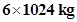and radius of the earth is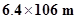.)

Ans.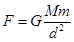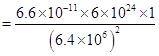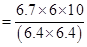= 9.81 N

4. The earth and the moon are attracted to each other by gravitational force. Does the earth attract the moon with a force that is greater or smaller or the same as the force with which the moon attracts the earth? Why?

Ans. The earth and the moon are attracted to each other by same gravitational force because for both of them formula to calculate force of attraction is the same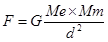d is also same for both.

5. If the moon attracts the earth, why does the earth not move towards the moon?

Ans. Earth does not move towards the moon because the mass of the moon is very small as compared to that of earth.

6. What happens to the force between two objects, if

(i) the mass of one object is doubled?

(ii) the distance between the objects is doubled and tripled?

(iii) the masses of both objects are doubled?

Ans. (i) The force between two objects will be doubled.

(ii) The force between two objects will become 1/4th and 1/9th of the present force.

(iii) The force between two objects will become four times the present force.

7. What is the importance of the universal law of gravitation?

Ans. The universal law of gravitation is important due to the following:

i) This law explains well the force that binds us to earth.

ii) This law describes the motion of planets around the sun.

iii) This law justifies the tide formation on earth due to moon and sun.

iv) This law gives the reason for the movement of the moon around the earth.

iv) This law gives the reason of keeping atmosphere close to the earth

8. What is the acceleration of free fall?

Ans. The acceleration of free fall is(on earth)

9. What do we call the gravitational force between the earth and an object?

Ans. Weight

10. Amit buys few grams of gold at the poles as per the instruction of one of his friends. He hands over the same when he meets him at the equator. Will the friend agree with the weight of gold bought? If not, why? [Hint: The value of is greater at the poles than at the equator.]

Ans. Since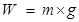and given in the question that value of g is greater at the poles than at the equator, hence the weight of the same amount of gold will be lesser at the equator than it was on the poles. Therefore, the friend will not agree with the weight of gold bought.

11. Why will a sheet of paper fall slower than one that is crumpled into a ball?

Ans. A greater surface area offers greater resistance and buoyancy same is true in the case of a sheet of paper that has a larger surface area as compared to paper crumpled into a ball. So a sheet of paper falls slower.

12. The gravitational force on the surface of the moon is only 1/6 as strong as gravitational force on the earth. What is the weight in newtons of a 10 kg object on the moon and on the earth?

Ans. The value of gravity on earth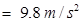the value of gravity on moon = 1/6th of earth = 9.8/6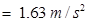the weight of the object on moon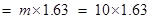= 16.3 N

the weight of the object on earth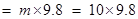= 98 N